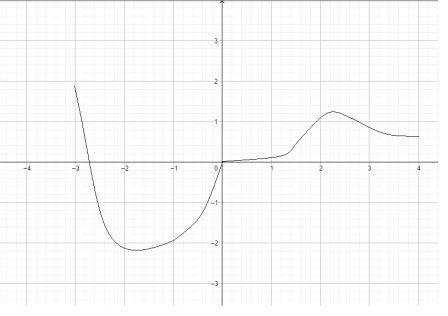# Consider a function f on the interval (-3,4) with the following properties: f is continuous on...

## Question:

Consider a function f on the interval (-3,4) with the following properties:

f is continuous on (-3,)

f(0) = 0

f'(1) = 0 and f'(3) = 0

f'(x) = -1 on the interval (-3,-1)

f'(x) > 0 and f"(x) < 0 on the interval (-1,0)

f'(x) > 0 on the interval (1,3)

f is concave down on (2,4) and concave up on (0,2)

a. f must have a local minimum at x = -1

True

False

Explain.

b. The absolute minimum of f is attained somewhere on (-3,4).

True

False

Explain.

c. There is an inflection point at x = 0.

True

False

Explain.

d. Sketch an example of a function f with the above properties.

## Concavity and Inflection

This problem is based on the detailed concept of the behavior of the function and its slope at different points. We will conduct a detailed mathematical analysis and observe the given sign changes to identify the points of maximum or minimum.

It is given that,

• The function is continuous in the interval {eq}\displaystyle (-3,4) {/eq}.
• {eq}\displaystyle f'(1)=0 \text{ and }f'(3)=0 {/eq}
• {eq}\displaystyle f'(x)=-1 {/eq} on the interval {eq}\displaystyle (-3,-1) {/eq}.
• {eq}\displaystyle f'(x)>0 \text{ and }f''(x)<0 {/eq} on the interval {eq}\displaystyle (-1,0) {/eq}
• {eq}\displaystyle f'(x)>0 {/eq} on the interval {eq}\displaystyle (1,3) {/eq}
• The function is concave down on {eq}\displaystyle (2, 4) {/eq} and concave up {eq}\displaystyle (0,2) {/eq}

Let us observe these given information closely.

It is given that {eq}\displaystyle f'(1)=0 \text{ and }f'(3)=0 {/eq}.

• We know that at critical points the first derivative of the function is either 0 or is undefined. Therefore, at {eq}\displaystyle x=1 \text{ and }x=3 {/eq} the function attains critical points. And thus, these could be points of maximum or minimum.

It is given that, {eq}\displaystyle f'(x) >0 {/eq}.

• Therefore, {eq}\displaystyle f(x) {/eq} is decreasing in the interval {eq}\displaystyle [-1,0] \cup [1,3] {/eq}.

It is also, given that the second derivative of the function changes its sign at {eq}\displaystyle x=0 {/eq}.

• Therefore, {eq}\displaystyle x=0 {/eq} must be a point of inflection.

Also, at {eq}\displaystyle x=-1 {/eq} the first derivative, {eq}\displaystyle f'(x) {/eq} changes its sign from negative to positive.

• Therefore, {eq}\displaystyle x=-1 {/eq} must be a point of minimum.
• Also, absolute minimum is attained somewhere between {eq}\displaystyle (-3,4) {/eq}.

• Hence, all the three statements are TRUE.

A rough sketch of such function is shown below: